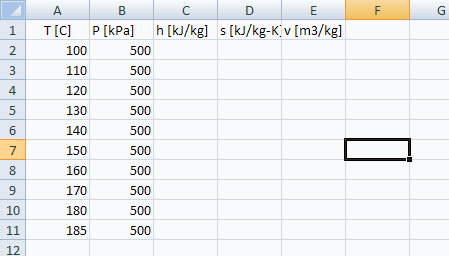Contents - Index

Using a Macro to Communicate with EXCEL or WORD

Communicating with EXCEL and WORD

Macro files can be used to set up a communication between EES and EXCEL or WORD.  A simple example is provided in the EXCEL1.emf macro file, which is provided in the USERLIB\EXAMPLES directory in the EES folder.  A listing of the macro file is as follows.

EXCEL.OPEN('C:\EES32\Userlib\Examples\EXCEL_WS1.xlsx')

EXCEL.COPY('A2:B11')

Paste Parametric 'Table 1'  R1 C1

SolveTable 'Table 1'

Copy ParametricTable 'Table 1' R1 C1 R10 C5

EXCEL.Range('A2:A2')

EXCEL.Paste

EXCEL.FileSaveAs('C:\EES32\Userlib\Examples\EXCEL_WS2.xlsx')

EXCEL.Quit

This macro is run from EES file TestEXCELMacro.ees, located in the USERLIB\EXAMPLES directory in the EES folder.  The Equations window for this file contains the following equations.

\$Include excel1.emf

R\$='R134a'

h=enthalpy(R\$,T=T,P=P)

s=entropy(R\$,T=T,P=P)

v=volume(R\$,T=T,P=P)

The EES file also has a Parametric table with 10 rows and columns for variables T, P, h, s, and v.

When the macro is played, EXCEL file 'C:\EES32\Userlib\Examples\EXCEL_WS1.xlsx' is opened.  The contents of this file are shown below.The first two columns containing temperature and pressure information are copied from EXCEL to the EES Parametric table.  EES then solves the table to calculate specific enthalpy, specific entropy and specific volume.  Then, the entire contents of the Parametric table are copied back to EXCEL and saved with a different file name.  EXCEL is then instructed to quit.  EXCEL is never visible during the execution of the macro.

Similar macro commands are available for communicating with WORD.  Note that EES can also communicate with EXCEL using Dynamic Data Exchange (DDE).  An example is provided here.